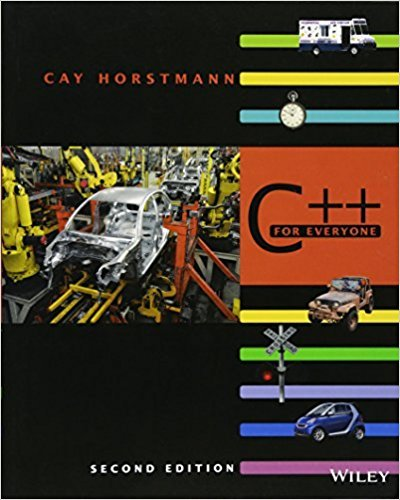×
Get Full Access to C++ For Everyone - 2 Edition - Chapter 8 - Problem R8.3
Get Full Access to C++ For Everyone - 2 Edition - Chapter 8 - Problem R8.3

×

# What happens if you try to open a file for reading that doesnt exist What happens ifyouISBN: 9780470927137 356

## Solution for problem R8.3 Chapter 8

C++ for Everyone | 2nd Edition

• Textbook Solutions
• 2901 Step-by-step solutions solved by professors and subject experts
• Get 24/7 help from StudySoup virtual teaching assistantsC++ for Everyone | 2nd Edition

4 5 1 287 Reviews
27
3
Problem R8.3

What happens if you try to open a file for reading that doesnt exist? What happens ifyou try to open a file for writing that doesnt exist?

Step-by-Step Solution:
Step 1 of 3

ENGR 121 B Lecture Notes for 9/26/2016 Spencer Kociba Summary of Lecture: how to classify, categorize, modify and conduct operations using matrices and vectors in MATLAB (which stands for Matrix Laboratory) MATRICES ● Matrix: stores a set of values of the same type ○ every value is an element ○ Matrix = m rows, n columns ● Creating Matrices ○ Ex. >> m=[ 1,4,6; 7,8,10; 2,7,15; 3,7,8] becomes a 4x3 matrix ■ 1,4,6 = 1st row, 7,8,10 = 2nd row, etc. ■ ; indicates the end of a row ■ MATLAB will show an error if your elements and the size of the matrix don’t add up ○ >>z=10*rand(3,5) becomes a 3x5 matrix with random numbers from (0,10) ○ >>z(1,2) refers to row 1, column 2 element ○ >>z(2,:) refers to all elements in row 2 ○ >>z(:,2) refers to all elements in column 2 ○ >>z([1,3], end) refers to 1st and 3rd row elements of the last column ● Matrix Multiplication ○ Maxtrix * Matrix = New Matrix ○ In A (n x m) and B (N x M), in order to multiply A*B, m=N. To multiply B*A, M=n ○ >>x=A*B ○ x.^(a) raises each element in matrix x by the power of a ● Matrix f

Step 2 of 3

Step 3 of 3

##### ISBN: 9780470927137

This textbook survival guide was created for the textbook: C++ for Everyone , edition: 2. This full solution covers the following key subjects: . This expansive textbook survival guide covers 10 chapters, and 515 solutions. Since the solution to R8.3 from 8 chapter was answered, more than 258 students have viewed the full step-by-step answer. The full step-by-step solution to problem: R8.3 from chapter: 8 was answered by , our top Engineering and Tech solution expert on 03/08/18, 08:39PM. C++ for Everyone was written by and is associated to the ISBN: 9780470927137. The answer to “What happens if you try to open a file for reading that doesnt exist? What happens ifyou try to open a file for writing that doesnt exist?” is broken down into a number of easy to follow steps, and 27 words.

## Discover and learn what students are asking

Chemistry: The Central Science : Periodic Properties of the Elements
?In the following reaction which sphere represents a metal and which represents a nonmetal? [Section 7.3

Statistics: Informed Decisions Using Data : Inference about Two Population Standard Deviations
?In Problems 9–14, assume that the populations are normally distributed. Test the given hypothesis. 14. s1 7 s2 at the a = 0.05 level of signi

Unlock Textbook Solution